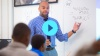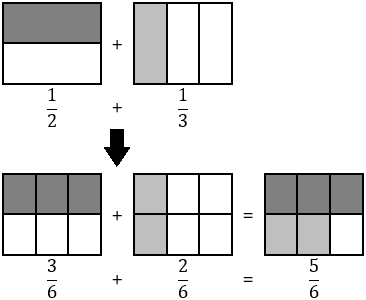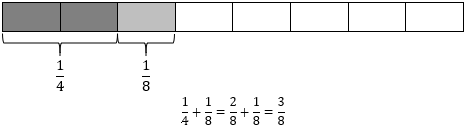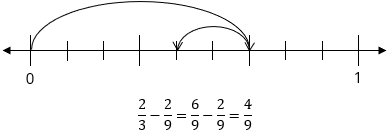# Addition and Subtraction of Fractions/Decimals

Students extend their computational work to include fractions and decimals, adding and subtracting numbers in those forms in this unit before moving to multiplication and division in subsequent units.

Math

Unit 4

## Unit Summary

In Unit 4, 5th grade students extend their computational work to include fractions and decimals, adding and subtracting numbers in those forms in this unit before moving to multiplication and division in subsequent units.

Students begin learning about fractions very early. In 1st grade and 2nd grade, students start to explore the idea of a fraction of a shape, visually representing halves, thirds, and fourths (1.G.3, 2.G.3). In 3rd grade, they build on this geometric idea of a fraction to develop an understanding of fractions as numbers themselves, using number lines as a representation to make that connection (3.NF.2). Students also start to compare fractions in special cases, including identifying equivalent fractions (3.NF.3). Then, in 4th grade, students extend their understanding of fraction equivalence and comparison. Students also add and subtract fractions with like denominators (4.NF.3) and multiply a fraction by a whole number (4.NF.4), work which they will rely on in this and the next unit.

Unit 4 starts with a refresher on work from 4th grade, starting with generating equivalent fractions and adding and subtracting fractions with like terms. While students are expected to already have these skills, they help to remind students that one can only add and subtract quantities with like units, as well as remind students how to regroup units (i.e., wholes). Then, students move toward adding and subtracting fractions with unlike denominators. They start with computing without regrouping, then progress to regrouping with small mixed numbers between 1 and 2, and then to regrouping with mixed numbers. Throughout this progression, students also progress from using more concrete and visual strategies to find a common denominator, such as constructing area models or number lines, toward more abstract ones like multiplying the two denominators together and using that product as the common denominator (5.NF.1). Then, students use this general method in more advanced contexts, including adding and subtracting more than two fractions, assessing the reasonableness of their answers using estimation and number sense (MP.1), and solving one-, two-, and multi-step word problems (5.NF.2), (MP.4). Then, the unit shifts its focus toward decimals, relying on their work in 4th grade of adding and subtracting decimal fractions (e.g., $$\frac{3}{10}+\frac{4}{100}=\frac{30}{100}+\frac{4}{100}=\frac{34}{100}$$) and their deep understanding that one can only add like units, including tenths and hundredths as those units, to add and subtract decimals (5.NBT.7). They use concrete models or drawings and strategies based on place value, properties of operations, and/or the relationship between addition and subtraction, relating the strategy to a written method and explain the reasoning used (MP.1). Students then apply this skill to the context of word problems to conclude the unit (MP.4).

As previously mentioned, students will explore the other operations, multiplication and division, of fractions and decimals in Unit 5 and Unit 6, including all cases of fraction and decimal multiplication and division of a unit fraction by a whole number and a whole number by a unit fraction (5.NF.3–7, 5.NBT.7). In 6th grade, students encounter the remaining cases of fraction division (6.NS.1) and solidify fluency with all decimal operations (6.NS.3). Students then rely on this operational fluency throughout the remainder of their mathematical careers, from fractional coefficients in functions to the connection between irrational numbers and non-repeating decimals.

Pacing: 18 instructional days (15 lessons, 2 flex days, 1 assessment day)

Fishtank Plus for Math

Unlock features to optimize your prep time, plan engaging lessons, and monitor student progress.## Assessment

The following assessments accompany Unit 4.

### Pre-Unit

Have students complete the Pre-Unit Assessment and Pre-Unit Student Self-Assessment before starting the unit. Use the Pre-Unit Assessment Analysis Guide to identify gaps in foundational understanding and map out a plan for learning acceleration throughout the unit.

### Mid-Unit

Have students complete the Mid-Unit Assessment after lesson 7.

### Post-Unit

Use the resources below to assess student understanding of the unit content and action plan for future units.

Expanded Assessment Package

Use student data to drive your planning with an expanded suite of unit assessments to help gauge students’ facility with foundational skills and concepts, as well as their progress with unit content.

## Unit Prep

### Intellectual Prep

Unit Launch

Prepare to teach this unit by immersing yourself in the standards, big ideas, and connections to prior and future content. Unit Launches include a series of short videos, targeted readings, and opportunities for action planning.#### Intellectual Prep for All Units

• Read and annotate “Unit Summary” and “Essential Understandings” portion of the unit plan.
• Do all the Target Tasks and annotate them with the “Unit Summary” and “Essential Understandings” in mind.
• Take the Post-Unit Assessment.

#### Unit-Specific Intellectual Prep

• When referred to fractions and decimals throughout Units 4--6, use unit language as opposed to “out of” or “point” language (e.g., $$\frac{3}{4}$$ should be described as “3 fourths” rather than “3 out of 4” and 1.2 should be described as “one and 2 tenths” rather than “one point two”). To understand why this is important for fractions (which can be extrapolated to decimals), read the following blog post: Say What You Mean and Mean What You Say by William McCallum on Illustrative Mathematics.
• Read the following table that includes models used in this unit.
 area model Example: Use an area model to solve $$\frac{1}{2}+\frac{1}{3}$$.tape diagram Example: Use a tape diagram to solve $$\frac{1}{4}+\frac{1}{8}$$.number line Example: Use a number line to solve $$\frac{2}{3}-\frac{2}{9}$$.### Essential Understandings

• Quantities cannot be added or subtracted if they do not have like units. Just like one cannot add 4 pencils and 3 bananas to have 7 of anything of meaning (unless one changes the unit of both to “objects”), the same applies for the units of fractions (their denominators) and the units of decimals (their places). This explains why one must find a common denominator to be able to add fractions with unlike denominators and why one must align corresponding places correctly (which in turn aligns the decimal points) when adding and subtracting decimals.
• “It is not necessary to find a least common denominator to calculate sums of fractions, and in fact the effort of finding a least common denominator is a distraction from understanding algorithms for adding fractions” (NF Progressions, p. 11).
• Similar to computational estimates with whole numbers, some computational estimates with fractions can be better than others, depending on what numbers are chosen to use in place of the actual values. Computational estimates can be too high or too low, depending on how the numbers were originally estimated, what computation is being performed, and where in the number sentence it’s located.
• "Because of the uniformity of the structure of the base-ten system, students use the same place value understanding for adding and subtracting decimals that they used for adding and subtracting whole numbers” (NBT Progression, p. 19).

### Materials

• Optional: Square-sized paper (2 per student) — See Lesson 4 Anchor Task 2 Notes for more information.

### Vocabulary

simplify

To see all the vocabulary for Unit 4, view our 5th Grade Vocabulary Glossary.

## Unit Practice

Word Problems and Fluency Activities

Access daily word problem practice and our content-aligned fluency activities created to help students strengthen their application and fluency skills.## Lesson Map

Topic A: Addition and Subtraction of Fractions

Topic B: Addition and Subtraction of Decimals

## Common Core Standards

Key

Major Cluster

Supporting Cluster

### Core Standards

#### Number and Operations in Base Ten

• 5.NBT.B.7 — Add, subtract, multiply, and divide decimals to hundredths, using concrete models or drawings and strategies based on place value, properties of operations, and/or the relationship between addition and subtraction; relate the strategy to a written method and explain the reasoning used.

#### Number and Operations—Fractions

• 5.NF.A — Use equivalent fractions as a strategy to add and subtract fractions.
• 5.NF.A.1 — Add and subtract fractions with unlike denominators (including mixed numbers) by replacing given fractions with equivalent fractions in such a way as to produce an equivalent sum or difference of fractions with like denominators. For example, 2/3 + 5/4 = 8/12 + 15/12 = 23/12. (In general, a/b + c/d = (ad + bc)/bd.)
• 5.NF.A.2 — Solve word problems involving addition and subtraction of fractions referring to the same whole, including cases of unlike denominators, e.g., by using visual fraction models or equations to represent the problem. Use benchmark fractions and number sense of fractions to estimate mentally and assess the reasonableness of answers. For example, recognize an incorrect result 2/5 + 1/2 = 3/7, by observing that 3/7 < 1/2.

• 4.NBT.B.4
• 5.NBT.A.1
• 5.NBT.A.4

• 4.NF.A.1
• 4.NF.A.2
• 4.NF.B.3
• 4.NF.C.5

• 4.OA.A.3

• 6.EE.B.7

• 6.NS.B.3
• 7.NS.A.1

### Standards for Mathematical Practice

• CCSS.MATH.PRACTICE.MP1 — Make sense of problems and persevere in solving them.

• CCSS.MATH.PRACTICE.MP2 — Reason abstractly and quantitatively.

• CCSS.MATH.PRACTICE.MP3 — Construct viable arguments and critique the reasoning of others.

• CCSS.MATH.PRACTICE.MP4 — Model with mathematics.

• CCSS.MATH.PRACTICE.MP5 — Use appropriate tools strategically.

• CCSS.MATH.PRACTICE.MP6 — Attend to precision.

• CCSS.MATH.PRACTICE.MP7 — Look for and make use of structure.

• CCSS.MATH.PRACTICE.MP8 — Look for and express regularity in repeated reasoning.

Unit 3

Shapes and Volume

Unit 5

Multiplication and Division of Fractions

## Request a Demo

See all of the features of Fishtank in action and begin the conversation about adoption.

Yes

No

### Any other information you would like to provide about your school?